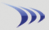Subscription & data-feed pricing Class schedule

Margin rates Stock & option commissions

Attention: Discussion forums are read-only for extended maintenance until further notice.

 Chaikin Money Flow (CMF) Topic Rating:Previous Topic · Next Topic Watch this topic · Print this topic ·
Bruce_L
 Posted : Tuesday, March 7, 2017 2:24:37 PMWorden Trainer

Joined: 10/7/2004
Posts: 65,138

# Chaikin Money Flow (CMF)

## Template (v17+)

AVG((2 * C - L - H) / (H - L - (H = L)) * V, x) / (AVGVx - (AVGVx = 0))

Where x is the period of the Chaikin Money Flow (CMF) indicator.

## Related Topics

-Bruce
Personal Criteria Formulas
TC2000 Support Articles
Aces4Me
 Posted : Thursday, June 8, 2017 7:55:14 AM
Registered User
Joined: 4/30/2015
Posts: 197

AVG((2 * C - L - H) / (H - L - (H = L)) * V, x) / (AVGVx - (AVGVx = 0))

AVG((2 * C - L - H) / (H - L - (H = L)) * V, 10) / (AVGV10 - (AVGV10 = 0))

Formula using the Above 10 Period for 10 period (5 period simple moving average)

Then formula for 10 Period CMF crossing UP its 5 SMA

and formula for 10 Period CMF crossing Down its 5 SMA

I AM ASSUMING that i can take the formula and change the 10 to any other period to have the formula work for other CMF periods?

Thanks

StockGuy
 Posted : Thursday, June 8, 2017 9:05:10 AMJoined: 9/30/2004
Posts: 9,187

I don't understand this "Formula using the Above 10 Period for 10 period (5 period simple moving average)".

Aces4Me
 Posted : Thursday, June 8, 2017 2:33:14 PM
Registered User
Joined: 4/30/2015
Posts: 197

ploting CMF , 10 period

Place a 5 period MA on the CMF 10

Then formula for 10 Period CMF crossing UP its 5 SMA

and formula for 10 Period CMF crossing Down its 5 SMA

StockGuy
 Posted : Thursday, June 8, 2017 2:45:34 PMJoined: 9/30/2004
Posts: 9,187

We can create the formula, but is there a reason creating the condition from the chart won't work? It's much more simple to do that. Just a couple of clicks.

StockGuy
 Posted : Thursday, June 8, 2017 3:03:50 PMJoined: 9/30/2004
Posts: 9,187

Crossing Up Mov Avg 5

AVG((2 * C - L - H) / (H - L - (H = L)) * V, 21) / (AVGV21 - (AVGV21 = 0)) > AVG((AVG((2 * C - L - H) / (H - L - (H = L)) * V, 21) / (AVGV21 - (AVGV21 = 0))),5) AND AVG((2 * C1 - L1 - H1) / (H1 - L1 - (H1 = L1)) * V1, 21) / (AVGV21.1 - (AVGV21.1 = 0)) < AVG((AVG((2 * C1 - L1 - H1) / (H1 - L1 - (H1 = L1)) * V1, 21) / (AVGV21.1 - (AVGV21.1 = 0))),5)

For crossing down, just reverse the > and <.

Aces4Me
 Posted : Thursday, June 8, 2017 3:36:56 PM
Registered User
Joined: 4/30/2015
Posts: 197

i will build a pcf with other condtions with it, into one formula

thanks

looks like 21 period so i change 21 to 10?

StockGuy
 Posted : Thursday, June 8, 2017 3:39:22 PM